Mobile QR Code1. (Program Studi Fisika, Universitas Pendidikan Indonesia, Jalan Dr. Setiabudhi 229, Bandung 40154, Indonesia)
2. (Physics of Electronic Material Research Division, Faculty of Mathematics and Natural Sciences, Institut Teknologi Bandung, Jalan Ganesa 10, Bandung 40132, Indonesia)
3. (Earth Physics and Physics of Complex Systems Research Division, Faculty of Mathematics and Natural Sciences, Institut Teknologi Bandung, Jalan Ganesa 10, Bandung 40132, Indonesia)

Armchair graphene nanoribbon, tunnel field-effect transistor, tunneling current, transfer matrix method, dirac-like equation

## I. INTRODUCTION

Miniaturization of silicon complementary metal-oxide-semiconductor (CMOS) devices has produced remarkable improvements to computer performance characteristics such as switching speed, density and functionality. However, the continuous reduction of silicon CMOS device dimensions has resulted in higher power consumption due to high leakage currents that degrade the switching ratio between on and off currents ($I_{ON}$/$I_{OFF}$) at low supply voltages. In order to extend computer performance, the search for new devices beyond CMOS has started, as discussed in recent review papers [1-3]. One such device, which can compete with or complement CMOS transistors, is the tunnel field-effect transistor (TFET), which uses a band-to-band tunnel for reducing $I_{OFF}$ in order to enhance $I_{ON}$/$I_{OFF}$ and make the subthreshold swing steeper [4-12]. The search for new materials with properties that can be better controlled by the electric field in TFETs continues. Si-based, III-V-based, and carbon nanotube materials are among them [13-18]. In addition, two-dimensional materials such as transition metal dichalcogenides (TMDs) as well as graphene provide other alternatives [19-25].

Graphene, which is composed of carbon atoms forming a hexagonal lattice, has interesting properties, such as one-atom thickness, planar geometry, zero band gap, very light effective mass, high carrier mobility, long phase coherence length, and its manufacturing process is compatible with conventional silicon technology [26,27]. Moreover, its band structure can be changed by limiting its size. When the width of graphene is much smaller than its length, it is called graphene nanoribbon (GNR) and it can be viewed as a one-dimensional electronic system [28,29]. There are two types of GNRs based on their edge shapes: armchair graphene nanoribbons (AGNRs) and zigzag graphene nanoribbons (ZGNRs). The structure of the energy band in graphene is determined using the tight binding approach [30-32]. Analysis of electron behavior around Dirac points or often called a low energy limit produces Dirac-like Hamiltonian [33,34] and its application to AGNR produces AGNR bandgap energy . The AGNRs can be either semiconducting or metallic, depending on their widths, while the ZGNRs have metallic properties for all widths [35,36]. By utilizing these properties, GNRs can be used as base material for logic devices such as field-effect transistors (FETs) and TFETs. Since the first proof of a graphene FET  further experimental studies have been conducted to obtain better devices [37-42].

Apart from the experimental studies, simulation and modelling of these devices has attracted researchers, either to explain experimental results or to develop future devices. Simpler atomistic semi-classical transport modelling and simulation have been performed by employing semi-classical Monte Carlo [43-45]. More rigorous atomic quantum transport modelling and simulation using the nonequilibrium Green function (NEGF)-based Schrödinger equation coupled with the Poisson equation have been implemented [46-50]. An improved model has been offered by utilizing the NEGF-based Dirac Hamiltonian coupled with the Poisson equation [51-53]. On the other hand, analytic quantum-mechanical modelling based on the Schrödinger equation with the WKB approach has also been applied [54-56]. However, an analytic model using the Dirac-like equation, which would be simpler than the NEGF-based Dirac Hamiltonian but more realistic than the Schrödinger equation, has not yet been applied to the devices. Electronic devices that have very recently been modelled analytically using the Dirac-like equation are an AGNR-based p-n junction , double gated FET  and a bipolar transistor .

In this paper, we report tunneling current characteristics in an AGNR-TFET that was modeled analytically under the Dirac-like equation. The transmittance was calculated by applying the transfer matrix method (TMM) and the tunneling current was calculated by using the Landauer formula. The TMM was utilized to obtain the tunneling current  and it was shown that the tunneling current calculated under the TMM is better than that under the WKB . Effects of drain voltage, gate voltage, width, and length of the AGNRs as well as oxide thickness on the tunneling current and subthreshold swing in AGNR-TFET are presented.

## II. THEORETICAL MODEL

The device structure of the n-channel AGNR-TFET in this paper is shown in Fig. 1(a). The armchair graphene nanoribbon (AGNR) type was utilized and doped it to be p+, n, and n+, which were utilized as source, channel and drain individually. The p and n-types of AGNR can be obtained by doping. Boron atoms (B) and Nitrogen (N) are appropriate dopants for graphene since B and N have similar structures to Carbon (C). The chemical vapor deposition (CVD) and electrothermal reaction are some experiments that have been reported to be successful by researchers to create p and n-types of graphene [62-65]. The potential profile of the AGNR-TFET was determined by solving the Dirac-like Hamilton equation, self-consistently with the Poisson equation. The finite difference method was used to discretize the Poisson equation and the Dirac-like Hamiltonian along the carrier transport direction, defined as the z direction ,

##### (1)
$H=v_{F}\left[\begin{array}{cc}{0} & {-i \hbar \partial_{z}-\hbar \partial_{x}} \\ {-i \hbar \partial_{z}+\hbar \partial_{x}} & {0}\end{array}\right]$

where $\hbar$ is the reduced Planck constant and $v_F$ is the Fermi velocity. Poisson’s equation solution by applying the surface potential method  used as guess potential. The iteration process between Dirac-like equation along with Poisson’s equation goes on up to the time that it reaches self-consistency. As Fig. 1(b) shows the conduction band of AGNR-TFET studied using the method of self-consistent at ON-state with 0.1 $V$ of drain voltage, and 0.1 $V$ of gate voltage, the AGNR width index N is 43 (around 5 nm), the gate length is 20 nm, and the thickness of the oxide is 1 nm . The AGNR width index N shows the number of atoms creating AGNR wide structure. These values associate with the AGNR width w according to $\mathrm{w}=\left(\mathrm{N}- 1\right)\frac{\sqrt{3}}{2}a_{cc}$ wherein $a_{cc}$ is the C-C bond length. AGNR is semiconducting for $N=3p +1$ and metallic for $N=3p$, where p is integer .

Fig. 1. (a) The n-channel AGNR-TFET structure, (b) The Energy band diagram of the AGNR-TFET at ON-state .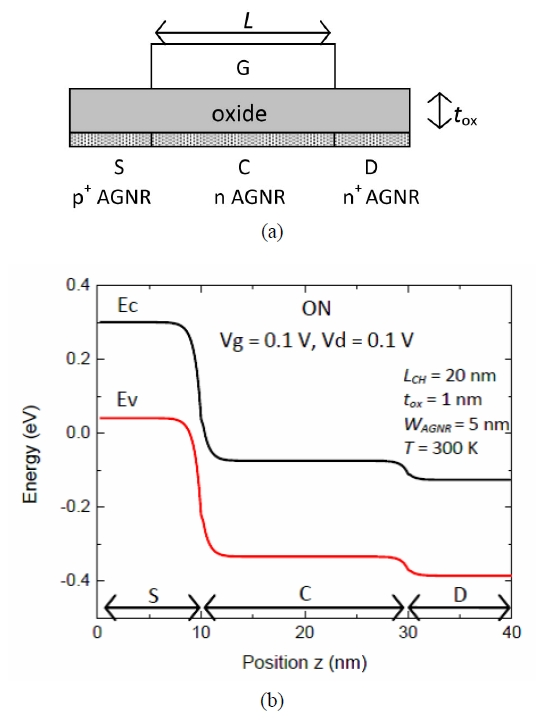The followings are the demonstration of the wave function solutions in each region which were acquired by using the Dirac-like equation along the carrier transport direction:

##### (2)
\begin{align} \begin{array}{lll} \psi _{1}=\frac{1}{\sqrt{2}}\left(\begin{array}{l} 1\\ \frac{\left| E- V_{1}\right| }{E- V_{1}}e^{i{\theta _{1}}} \end{array}\right)e^{i{k_{1}}z+i{k_{n}}x}+\frac{B_{1}}{\sqrt{2}}\left(\begin{array}{l} 1\\ - \frac{\left| E- V_{1}\right| }{E- V_{1}}e^{- i{\theta _{1}}} \end{array}\right)e^{- i{k_{1}}z+i{k_{n}}x},\,\,\,\,\,\,\,\,\,\,\,\,\,\,\,\,\,\,\,\,\,\,\,\,\,\,\,\,\,\,\,\,\,\,\,\,\,\,\,\,\,\,\,\,\,\,\, & & z\leq 0\\ \psi _{S,C,D}=\frac{A_{S,C,D}}{\sqrt{2}}\left(\begin{array}{l} 1\\ \frac{\left| E- V_{S,C,D}\right| }{E- V_{S,C,D}}e^{i{\theta _{SCD}}} \end{array}\right)e^{i{k_{SCD}}z+i{k_{n}}x}+\frac{B_{S,C,D}}{\sqrt{2}}\left(\begin{array}{l} 1\\ - \frac{\left| E- V_{S,C,D}\right| }{E- V_{S,C,D}}e^{- i{\theta _{SCD}}} \end{array}\right)e^{- i{k_{SCD}}z+i{k_{n}}x} & & 0<z\leq 2L\\ \psi _{2}=\frac{A_{2}}{\sqrt{2}}\left(\begin{array}{l} 1\\ \frac{\left| E- V_{2}\right| }{E- V_{2}}e^{i{\theta _{2}}} \end{array}\right)e^{- i{k_{2}}z+i{k_{n}}x}\,\,\,\,\,\,\,\,\,\,\,\,\,\,\,\,\,\,\,\,\,\,\,\,\,\,\,\,\,\,\,\,\,\,\,\,\,\,\,\,\,\,\,\,\,\,\,\,\,\,\,\,\,\,\,\,\,\,\,\,\,\,\,\,\,\,\,\,\,\,\,\,\,\,\,\,\,\,\,\,\,\,\,\,\,\,\,\,\,\,\,\,\,\,\,\,\,\,\,\,\,\,\,\,\,\,\,\,\,\,\, & & z>2L, \end{array} \end{align}

The aforementioned formula can be explained where $B_1$, $A_{S}$, $B_{S}$, $A_{C}$, $B_{C}$, $A_{D}$, $B_{D}$ and $A_{2}$ are constants, $V$ is the potential energy, $L$ acts as the channel length (the source length = the drain length = $L/2$), $k_{1,S,C,D,2}=$ $\sqrt{\left(E- V_{1,S,C,D,2}\right)^{2}/\left(\mathrm{\hslash }^{2}v_{F}^{2}\right)- k_{n}^{2}}$, $k_n$ take action as the transverse momentum, and, $\theta _{1,S,C,D,2}=\tan ^{- 1}\left(k_{n}/k_{1,S,C,D,2}\right)$. Indices $S$, $C$, and $D$ point out the region in source, channel, and drain respectively, while indices 1 and 2 shows the region out of AGNR-TFET, in this case is metal. The transmittance was studied by utilizing the TMM following the method in Refs. [57-61]. The acquired transmittance was then exerted to compute the tunneling currents by using the Landauer formula,

##### (3)
\begin{equation} Id=\frac{2g_{v}e}{h}\int _{E_{1}}^{E_{2}}\left[f_{S}\left(E\right)- f_{D}\left(E\right)\right]T\left(E\right)dE \end{equation}

where $f_{S}(E)$ and $f_{D}(E)$ are the Fermi-Dirac energy distribution functions for electrons in the source and the drain, respectively, $g_r$ is the degeneration of GNR ($g_r= 1$), $h$ is the Planck constant, $E_{1}$ and $E_{2}$ are limit for energy integration and $T(E)$ is the electron transmittance.

## III. CALCULATED RESULTS AND DISCUSSION

Fig. 2 indicates the study result of implementation of Dirac-like equation on the tunneling currents (Id) as a function of drain voltage (Vd) for various gate voltages (Vg). For comparison, we also show the tunneling current calculated by using the Schrödinger equation through WKB approximation . AGNR channel length L, AGNR width index N, oxide thickness tox, and the temperature were 20 nm, 43 (about 5 nm), 1 nm, and 300 K, respectively. The tunneling current studied by both equations pointed out the same features and the metal-oxide-semiconductor field-effect transistor (MOSFET) type behaviour was revealed. It was shown that the tunneling currents initially increase with the drain voltage and then reach saturation. When gate voltage was added, the conduction band in the channel decreased, so the valence band in the source was higher than the conduction band in the channel. Electrons can tunnel from the valence band at the source to the conduction band in the channel. The tunneling current increased significantly when the gate voltage was increased. Supposing that the drain voltage is boosted constantly along with the gate voltage fixed, the amount of electrons in that tunnel remain the same as the valence band at the source and the conduction band in the channel do not experience alteration with the escalation of the drain voltage. In this example, the current was somewhat steady and saturation took place. Moreover, the tunneling current that was examined by implementing the Dirac-like equation was lower than that acquired by using the Schrödinger equation.

Fig. 2. The tunneling current as a function of drain voltage for various gate voltages calculated using the Schrödinger equation through WKB approximation and the Dirac-like equation through TMM.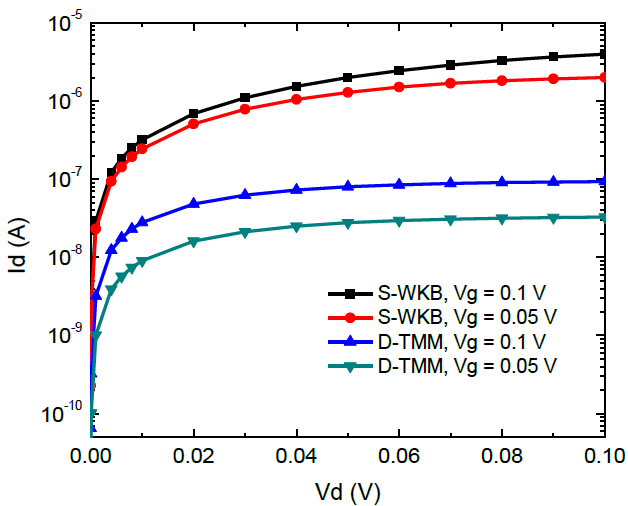Fig. 3(a) and (b) show the tunneling current as a function of gate voltage calculated using the Schrödinger equation through WKB approximation and the Dirac-like equation through TMM respectively. It can be seen from Fig. 3(a) that the dependence of tunneling current on gate voltage for a fixed drain voltage was linear. We had difficulty determining the threshold voltage of the device. However, the dependence of the tunneling current on the gate voltage for a fixed drain voltage calculated by using the Dirac-like equation through TMM was exponential for low gate voltages. The threshold voltage of the device could easily be determined. The threshold voltage of the device was about 0.01 V.

Fig. 3. The tunneling current as a function of gate voltage calculated using (a) the Schrödinger equation through WKB approximation, (b) the Dirac-like equation through TMM.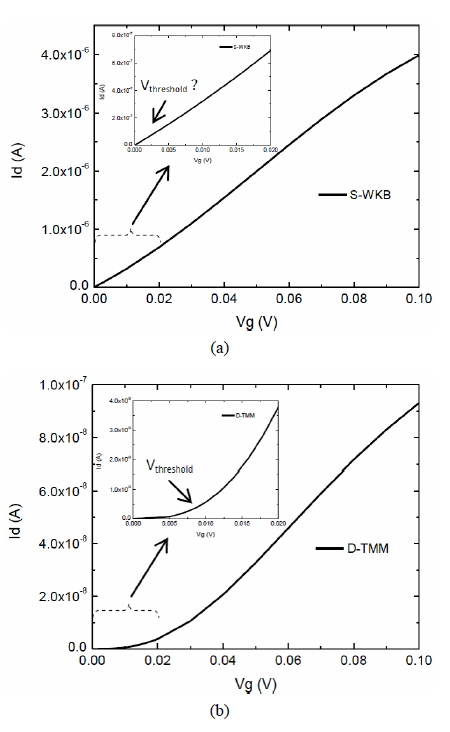Current density as a function of gate voltage is presented in Fig. 4. It also shows the subthreshold swing characteristic of the device. The current density calculated by using the Schrödinger equation through WKB approximation was higher than that obtained by the Dirac-like equation through TMM. The subthreshold swing calculated using the Schrödinger equation through WKB approximation was 0.19 $mV/dec$ , while the subthreshold swing calculated by using the Dirac-like equation was approximately 5 $mV/dec$. We can get the subthreshold swing lower than 5 $mV/dec$ by taking a smaller threshold voltage as the result of the calculations using the Schrödinger equation.

Fig. 4. The tunneling currents density as a function of gate voltage calculated using the Schrodinger equation through WKB approximation and the Dirac-like equation through TMM.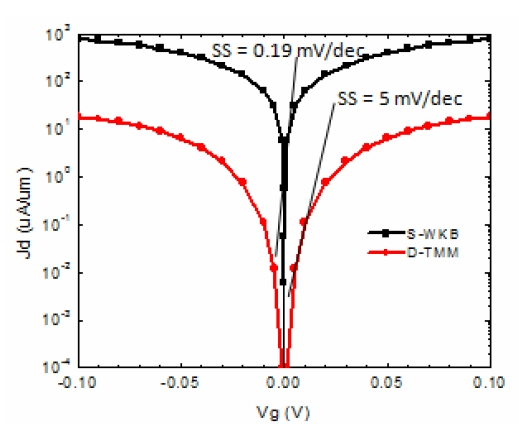Fig. 5(a) demonstrates the dependence of the tunneling current on the drain voltage for different AGNR widths index. AGNR channel length L, oxide thickness tox, the temperature and the gate voltage are 20 nm, 1 nm, 300 K, and 0.1 $V$ respectively. The AGNR width index variations were 25, 34, 43 and 52 related to AGNR width about 3 nm, 4 nm, 5 nm and 6 nm. It was shown that the tunneling current increased with increasing AGNR width. Fig. 5(b) shows the tunneling current as a function of gate voltage for various AGNR widths on a logarithmic scale. The effect of AGNR width on subthreshold swing can be seen in Fig. 5(b). The insert explains the differences in subthreshold swing for variations in width. The subthreshold swing increased with increasing AGNR width. This can be understood from the fact that the AGNR band-gap is inversely proportional to the AGNR width [35,36]. As the AGNR width increases, the AGNR band-gap decreases, so more electrons enter the conduction band and the tunneling currents increases. This condition also affects the off current ($I_{OFF}$) and the on current ($I_{ON}$) of the device. The $I_{OFF}$ increase is larger than the $I_{ON}$ increase (on current). Consequently, the $I_{ON}$/$I_{OFF}$ ratio decreases and increases the subthreshold swing.

Fig. 5. The tunneling currents as a function of (a) drain voltage, (b) gate voltage for various AGNR widths index.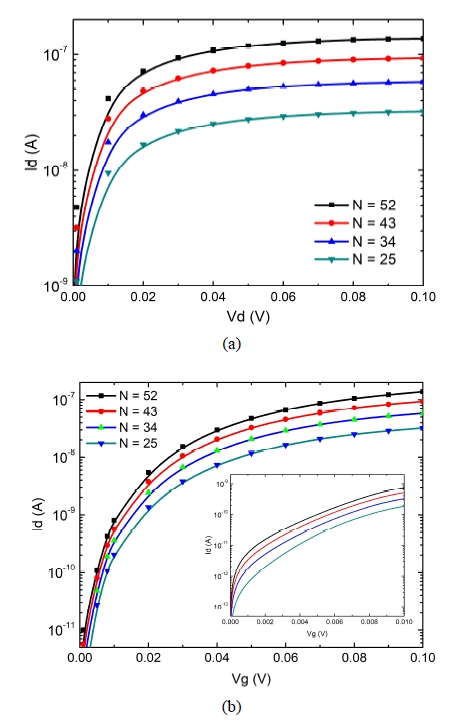The dependence of the tunneling current on the drain voltage for different AGNR lengths is given in Fig. 6(a). AGNR width index N, oxide thickness $t_{ox}$, the temperature and the gate voltage are 43, 1 nm, 300 K, and 0.1 $V$ respectively. It can be seen that the tunneling current increased with decreasing AGNR length. Fig. 6(b) represents tunneling current as a function of the gate voltage for various AGNR lengths on a logarithmic scale. The insert in Fig. 6(b) displays the different subthreshold swings for various AGNR lengths. The off current $I_{\mathrm{OFF}}$ decreased when the AGNR length increased so the $I_{\mathrm{ON}}$/$I_{\mathrm{OFF}}$ ratio increased and the subthreshold swing decreased.

Fig. 7(a) explores the tunneling current as a function of drain voltage for different oxide thicknesses. AGNR channel length L, AGNR width w, the temperature and the gate voltage are 20 nm, 43, 300 K, and 0.1 $V$ respectively. Fig. 7(b) presents tunneling current as a function of gate voltage for various oxide thicknesses on a logarithmic scale. It can be observed that the tunneling current of the AGNR-TFET increased with decreasing oxide thickness. The oxide thickness affects the gate voltage control on the channel of the device. This also demonstrates that the oxide thickness is inversely proportional to the conductivity of the AGNR-TFET. The dependence of the tunneling current on the oxide thickness has a similar characteristic to MOSFET, nanowire FET and CNT-FET . The effect of oxide thickness on the subthreshold swing of the device is depicted in the insert of Fig. 7(b). It can be seen that the subthreshold swing of the device increased with increasing oxide thickness.

Fig. 6. The tunneling currents as a function of (a) drain voltage, (b) gate voltage for various channel length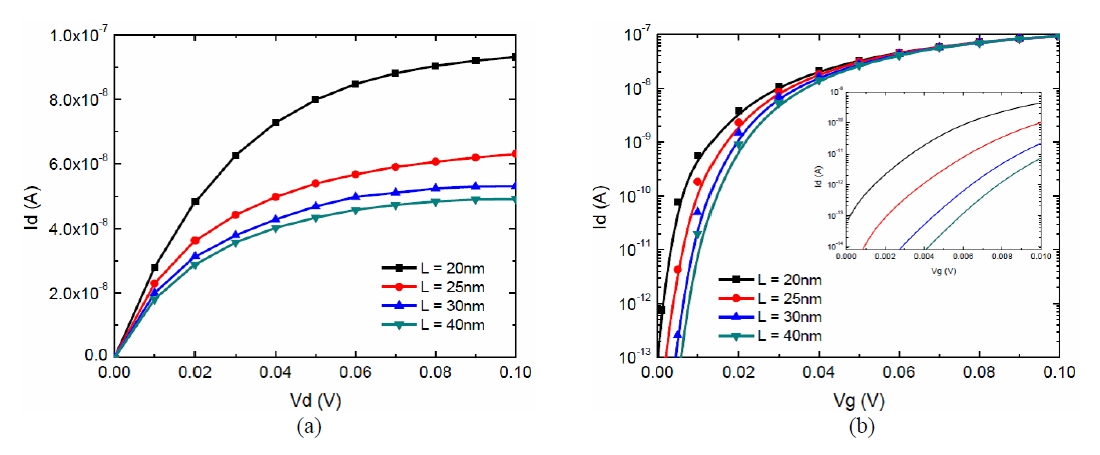Fig. 7. The tunneling currents as a function of (a) drain voltage, (b) gate voltage for various oxide thicknesses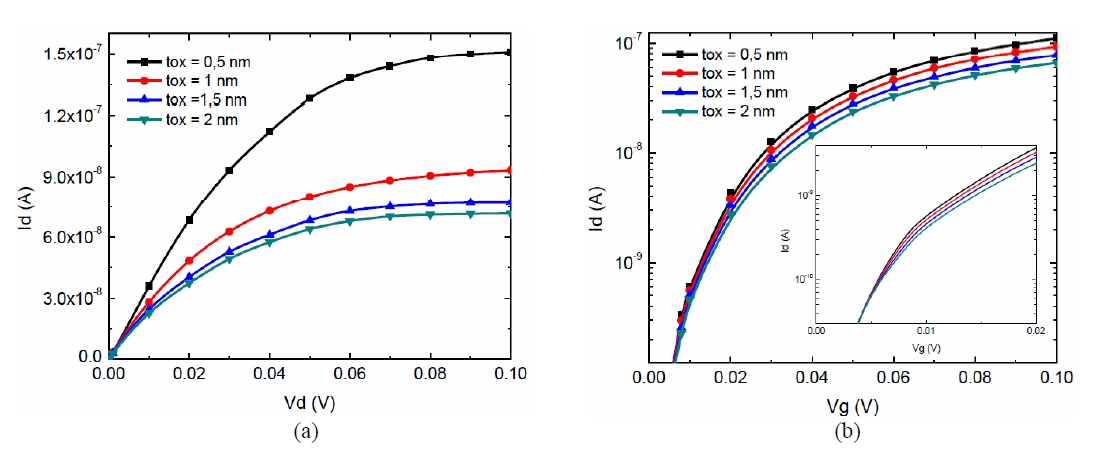The effects of temperature to the tunneling current AGNR-TEFT depicted on Fig. 8(a) and (b). Fig. 8(a) shows tunneling current in linear scale as a drain voltage function to different temperature and Fig. 8(b) shows tunneling current in logarithmic scale as a gate function with different temperature. At this point, AGNR width index, channel length, the thickness of the oxide continuously is 43, 20 nm and 1 nm. Gate voltage and source voltage is still at 0.1 V. Tunneling current will increase as the temperature decrease. Mobility factors may hold a dominant factor to explain the dependence tunneling current to AGNR-TEFT. Charge carrier mobility in graphene is quiet high, it is around 230.000 cm$^{2 }$V$^{{-}1}$ s$^{{-}1}$ on 5K  and 185.000 cm$^{2 }$V$^{{-}1}$ s$^{{-}1}$ on 100K . When temperature increases electron mobility decreases due to grating vibration keeps climbing, therefore the probabilities of electron scattered by grating will increase. The dependence of tunneling current to temperature for electronic device based AGNR have been reported by other researchers as well [56,70].

Fig. 8. The tunneling currents as a function of (a) drain voltage, (b) gate voltage for various temperature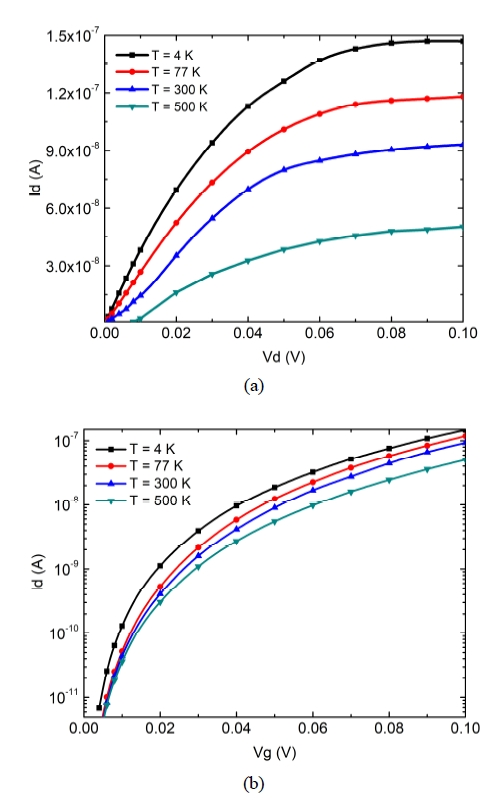## IV. CONCLUSION

In the present paper, the characteristics of an armchair graphene nanoribbon tunnel field-effect transistor (AGNR-TFET) were modeled. The Dirac-like equation was used to explore the characteristics of the device. The electron transmittance and tunneling current were derived by employing the transfer matrix method (TMM) and the Landaur formula. It was found that the tunneling current calculated by using the Dirac-like equation was lower than that obtained using the Schrödinger equation. The threshold voltage and the subthreshold swing of the device calculated by using the Dirac-like equation were about 0.01 $V$ and 5 $mV/dec$ respectively. It was also found that the AGNR-TFET geometry, i.e. width and length of GNR and oxide thickness, affects the tunneling current and the subthreshold of the device. The tunneling current increased as the width of AGNR and the oxide thickness increased, while it decreased with increasing AGNR length and temperature. The subthreshold swing increased with increasing AGNR width while an increase of the AGNR length caused the subthreshold swing to decrease. The subthreshold swing of the device increased with increasing oxide thickness.

### ACKNOWLEDGMENTS

This work was financially supported by “Hibah Penelitian Berbasis Kompetensi” Research Grants, Direktorat Riset dan Pengabdian Masyarakat, Direktorat Jenderal Penguatan Riset dan Pengembangan, Kementrian Riset, Teknologi, dan Pendidikan Tinggi Republik Indonesia in the fiscal year 2018.

### REFERENCES

1
Seabaugh A. C., Zhang Q., Dec 2010, Low voltage tunnel transistors for beyond CMOS logic, Proc. IEEE 98, pp. 2095-21102
Bernstein K., 2010, Device and architectures outlook for beyond CMOS switches, Proc. IEEE 98, pp. 2169-21843
Schwierz F., 2010, Graphene transistors, Nature Nanotech, Vol. 5, pp. 487-496.4
Datta S., Liu H., Narayanan V., 2014, Tunnel FET Technology: A Reliability Perspective, Microelectronis Reability, Vol. 54, No. , pp. 861-874.5
Appenzeller J., 2004, Band-to-Band Tunneling in Carbon Nanotube Field-Effect Transistors, Physics Review Letter, Vol. 93, No. 1968056
Zhu Y., Hudait M. K., 2013, Low-Power Tunnel Field Effect Transistors Using Mixed As and Sb Based Heterostructures, Nanotechnology Review, Vol. 2, pp. 637-6787
Mohata D., 2012, Demonstration of Improved Heteroepitaxy, Scaled Gate Stack and Reduced Interface States Enabling Heterojunction Tunnel FETs with High Drive Current and High on-off Ratio, VLSI technology (VLSIT) Symposium, pp. 53-448
Zhao H., 2011, Improving the On-Current of In07Ga03As Tunneling Field-Effect-Transistors by p++/n+ Tunneling Junction, Applied. Phys. Lett., Vol. 98, No. , pp. -9
Noguchi M., 2015, High $I_{ON}$/$I_{OFF}$ and low subthreshold slope planar-type InGaAs tunnel field effect transistors with Zn-diffused source junctions, Journal of Applied Physics, Vol. 118, No. 4, pp. 045712-10
Tomioka K., Fukui T., 2014, Current increment of tunnel field-effect transistor using InGaAs nanowire/Si heterojunction by scaling of channel length, Appl. Phys. Lett., Vol. 104, No. 07350711
Zhan Z., 2012, A tunnel-induced injection field-effect transistor with steep subthreshold slope and high on-off current ratio, Appl. Phys. Lett., Vol. 100, No. 11351212
Tomioka K., Fukui T., 2011, Tunnel field-effect transistor using InAs nanowire/Si heterojunction, Applied Physics Letter, Vol. 98, No. 08311413
Choi W. Y., 2007, Tunneling field-effect transistors (TFETs) with subthreshold swing (SS) less than 60 $mV/dec$, IEEE Electron Device Lett., Vol. 28, No. 8, pp. 743-74514
Bhuwalka K. K., Schulze J., Eisele I., 2004, Performance enhancement of vertical tunnel field-effect transistor with SiGe in the p+ layer, Jpn. J. Appl. Phys., Vol. 43, pp. 4073-407815
Wang L., 2010, Design of Tunneling Field-Effect Transistors Based on Staggered Heterojunctions for Ultralow-Power Applications, IEEE Electron Device Lett., Vol. 31, No. 5, pp. 431-43316
Moselund K. E., 2012, InAs-Si Nanowire Heterojunction Tunnel FETs, IEEE Electron Device Lett., Vol. 33, No. 10, pp. 1453-145517
Appenzeller J., 2005, Comparing carbon nanotube transistors-The ideal choice: A novel tunneling device design, IEEE Trans. Electron Devices, Vol. 52, No. 12, pp. 2568-257618
Poli S., 2008, Computational study of the ultimate scaling limits of CNT tunneling devices, IEEE Trans. Electron Devices, Vol. 55, No. 1, pp. 313-32119
Radisavljevic B., 2011, Single-layer MoS2 transistors, Nature Nanotech., Vol. 6, pp. 147-15020
Fang H., 2012, High Performance Single Layered WSe2 p-FETs with Chemically Doped Contacts, Nano Lett., Vol. 12, No. 7, pp. 3788-379221
Butler. S. Z., 2013, Progress, challenges, and opportunities in two-dimensional materials beyond graphene, ACS nano, Vol. 7, No. 4, pp. 2898-292622
Fiori G., 2014, Electronics based on two-dimensional materials, Nature Nanotech., Vol. 9, pp. 768-77923
Jariwala D., 2014, Emerging Device Applications for Semiconducting Two-Dimensional Transition Metal Dichalcogenides, ACS Nano, Vol. 8, No. 2, pp. 1102-112024
Britnell L., 2012, Field-effect tunneling transistor based on vertical graphene heterostructures, Science, Vol. 335, No. 6071, pp. 947-95025
Schwierz F., 2013, Graphene transistors: status, prospects, and problems, Proc. IEEE, Vol. 101, No. 7, pp. 1567-158426
Novoselov K. S., 2004, Electric field effect in atomically thin carbon films, Science, Vol. 306, No. 5696, pp. 666-66927
Berger C., 2006, Electronic confinement and coherence in patterned epitaxial graphene, Science, Vol. 312, No. 5777, pp. 1191-119628
Li X., 2008, Chemically derived, ultrasmooth graphene nanoribbon semiconductors, Science, Vol. 319, No. 5867, pp. 1229-123229
Raza H., Kan E. C., 2008, Armchair graphene nanoribbons: Electronic structure and electric-field modulation, Physical Review B, Vol. 77, No. 24, pp. 24543430
Wu Y., 2011, Conductance of Graphene Nanoribbon Junctions and the Tight Binding Model, Nanoscale Research Letters, Vol. 6, No. 6231
Bena C., Motambux G., 2009, Remarks on The Tight-Binding Model of Graphene, New Journal of Physics, Vol. 11, No. 09500332
Gruneis A., 2008, Tight-Binding Description of The Quasiparticle Dispersion of Graphite and Few-Layer Graphene, Physical Review B, No. 78, pp. 20542533
Gruneis A., 2009, Angle-Resolved Photoemission Study of The Graphite Intercalation Compound KC8: A Key to Graphene, Physical Review B, Vol. 80, No. 07543134
Katsnelson M. I., Novoselov K. S., Geim A. K., 2006, Chiral tunnelling and the Klein paradox in graphene, Nature Phys., Vol. 2, No. , pp. 620-62535
Brey L., Fertig H. A., 2006, Electronic states of graphene nanoribbons, Phys. Rev. B, Vol. 73, No. 23541136
Son Y. W., Cohen M. L., Loui S. G., 2006, Energy gaps in graphene nanoribbons, Phys. Rev.Lett., Vol. 97, No. 21680337
Chen Z., 2007, Graphene nano-ribbon electronics, Physica E: Low-dimensional Systems and Nanostructures, Vol. 40, No. 2, pp. 228-23238
Wang X., 2008, Room-temperature all-semiconducting sub-10-nm graphene nanoribbon field-effect transistors, Phys. Rev. Lett., Vol. 100, No. 20, pp. 20680339
Xia F., 2010, Graphene field-effect transistors with high on/off current ratio and large transport band gap at room temperature, Nano Lett., Vol. 10, No. 2, pp. 715-71840
Son J. G., 2013, Sub-10 nm Graphene Nanoribbon Array Field-Effect Transistors Fabricated by Block Copolymer Lithography, Advanced Materials, Vol. 25, No. 34, pp. 4723-472841
Zschieschang U., 2015, Electrical Characteristics of Field-Effect Transistors based on Chemically Synthesized Graphene Nanoribbons, Advanced Electronic Materials, Vol. 1, No. 342
Hwang W. S., 2015, Graphene nanoribbon field-effect transistors on wafer-scale epitaxial graphene on SiC substrates, APL Materials, Vol. 3, No. 1, pp. 01110143
David J. K., Register L. F., Banerjee S. K., 2012, Semiclassical Monte Carlo Analysis of Graphene FETs, IEEE Trans. Electron Dev., Vol. 59, No. 4, pp. 976-98244
Paussa A., 2014, Simulation of the Performance of Graphene FETs With a Semiclassical Model, Including Band-to-Band Tunneling, IEEE Trans. Electron Dev., Vol. 61, No. 5, pp. 1567-157445
Pugnaghi C., 2014, Semianalytical quantum model for graphene field-effect transistors, J. Appl. Phys., Vol. 116, pp. 11450546
Fiori G., Ianaccoce G., 2007, Simulation of Graphene Nanoribbon Field-Effect Transistors, IEEE Electron Dev. Lett., Vol. 28, No. 8, pp. 760-76247
Zhao P., Chauhan J., 2009, Computational Study of Tunneling Transistor Based on Graphene Nanoribbon, Nano Lett., Vol. 9, pp. 684-68048
Noei M., Moradinasab M., Fathipour M., 2012, A computational study of ballistic graphene nanoribbon field effect transistors, Physica E, Vol. 44, pp. 1780-178649
Mohamadpour H., Asgari A., 2012, Graphene nanoribbon tunneling field effect transistors, Physica E, Vol. 46, pp. 270-27350
Yousefi R., 2013, A computational study on electrical characteristics of a novel band-to-band tunneling graphene nanoribbon FET, Superlattices & Microstructures, Vol. 60, pp. 169-17851
Chin S-K., 2010, Device Physics and Characteristics of Graphene Nanoribbon Tunneling FETs, IEEE Trans. Electron Dev., Vol. 57, No. 11, pp. 3144-315252
Lam K-T., 2010, A Simulation Study of Graphene-Nanoribbon Tunneling FET With Heterojunction Channel, IEEE Electron Dev. Lett., Vol. 31, No. 6, pp. 555-55753
Chauhan J., Guo J., 2011, Assessment of high-frequency performance limits of graphene field-effect transistors, Nano Res., Vol. 4, No. 6, pp. 571-57954
Zhang Q., 2008, Graphene Nanoribbon Tunnel Transistors, IEEE Electron Dev. Lett., Vol. 29, No. 12, pp. 1344-134655
Sarkar D., Krall M., Banerjee K., 2010, Electron-hole duality during band-to-band tunneling process in graphene-nanoribbon tunnel-field-effect-transistors, Appl. Phys. Lett., Vol. 97, No. 26, pp. 26310956
Putro C. B. S., 2014, A Theoretical Model of Band-to-band Tunneling Current in an Armchair Graphene Nanoribbon Tunnel Field-Effect Transistor, Adv. Mater. Res., Vol. 896, pp. 371-37457
Suhendi E., 2014, Simulation of Dirac Tunneling Current of an Armchair Graphene Nanoribbon-Based p-n Junction Using a Transfer Matrix Method, Adv. Mater. Res., Vol. 974, pp. 205-20958
Suhendi E., 2014, Simulation of Drain Current of Double Gated Armchair Graphene Nanoribbon Field-Effect Transistor by Solving Dirac, Journal of Physics: Conf. Ser., Vol. 539, No. 01202059
Suhendi E., 2015, Modeling of Dirac Electron Tunneling Current in Bipolar Transistor Based on Armchair Graphene Nanoribbon Using a Transfer Matrix Method, Adv. Com. Sci. Res., Vol. 5, pp. 164-16660
Abdolkader T. M., Hassan M. H., Fikry W., 2004, Solution of Schrödinger equation in double-gate MOSFETs using transfer matrix method, Electron Lett., Vol. 40, No. 20, pp. 1307-130861
Shangguan W. Z., 2005, Compact gate-current model based on transfer-matrix method, J. Appl. Phys., Vol. 97, No. 12370962
Cattelan M., 2013, Microscopic view on a chemical vapor deposition route to boron-doped graphene nanostructures, Chem Mater, Vol. 25, pp. 1490-149563
Gebhardt J., 2013, Growth and electronic structure of boron-doped graphene, Phys Rev B, Vol. 87, No. 15543764
Tang Y. B., 2012, Tunable band gaps and p-type transport properties of boron-doped graphenes by controllable ion doping using reactive microwave plasma, ACS Nano, Vol. 6, No. 3, pp. 1970-197865
Wei D., 2009, Synthesis of N-doped graphene by chemical vapor deposition and its electrical properties, Nano Letters, Vol. 9, No. 5, pp. 1752-175866
Suhendi E., 2019, Comparison of tunneling currents in graphene nanoribbon tunnel field effect transistors calculated using Dirac-like equation and Schrdinger's equation, J. Semicond., Vol. 40, No. 6, pp. 06200267
Tiwari M., 2015, Impact of Oxide Thickness on Gate Capacitance, Drain Current and Transconductance - A Comprehensive analysis on MOSFET, Nanowire FET and CNTFET Devices, Int. Jou. for Research in Emerging Science and Technology, Vol. 2, pp. 73-8568
Bolotin K. I., 2008, Ultrahigh Electron Mobility in Suspended Graphene, Solid State Communication, Vol. 146, pp. 351-35569
Du X., 2008, Approaching Ballistic Transport in Suspended Graphene, Nature Nanotechnology, Vol. 3, pp. 491-49570
Jena D., 2008, Zener Tunneling in Semiconducting Nanotube and Graphene Nanoribbon p−n Junctions, Appl. Phys. Lett., Vol. 93, pp. 112106## Author

Endi Suhendi is an associate professor at Departemen Pendidikan Fisika, Universitas Pendidikan Indonesia, Bandung, Indonesia.

He received his Doctoral degree from Departmen Fisika, Institut Teknologi Bandung, Indonesia, in 2015.

His current research interests are graphene-based nanoelectronic devices and sensors.

Lilik Hasanah received her Doctoral degree from Departmen Fisika, Institut Teknologi Bandung, Indonesia, in 2008.

She is an associate professor at Departemen Pendidikan Fisika, Universitas Pendidikan Indonesia, Bandung, Indonesia.

Her current research interests are semiconductor materials and devices.

Fatimah Arofiati Noor received her B.S., M.S and doctoral degree in Physics from Institut Teknologi Bandung Indonesia, in 2004, 2006 and 2010, respectively.

She is an associate professor at Physics Department, Faculty of Mathematics and Natural Sciences, Institut Teknologi Bandung, Indonesia.

Her current research interests are semiconductor materials and devices.

Neny Kurniasih received her B.S. and M.S degree in Physics from Institut Teknologi Bandung Indonesia, in 1985 and 1989.

She received Ph.D degree in global environment engineering from Kyoto University, Japan, in 2001.

She is an associate professor at Departemen Fisika, Institut Teknologi Bandung, Indonesia.

Her current research interests is quantitative non-destructive evaluation of flaws within structures using time-domain boundary integral equation method.

Khairurrijal received his B.S. and M.S degree in Physics from Institut Teknologi Bandung Indonesia, in 1989 and 1993.

He received Doctor of engineering from Graduate Program of Electrical Engineering, Graduate School of Engineering, Hiroshima University, Japan, in 2000.

He is a professor at Departemen Fisika, Institut Teknologi Bandung, Indonesia.

He has authored and co-authored over 30 international journals and conference papers.

His current research interests are electronic materials, devices and instrumentation.